# How to use Excel COUNTIFS and COUNTIF with multiple criteria

The tutorial explains how to use COUNTIFS and COUNTIF formulas with multiple criteria in Excel based on AND as well as OR logic. You will find a number of examples for different data types - numbers, dates, text, wildcard characters, non-blank cells and more.

Of all Excel functions, COUNTIFS and COUNTIF are probably most often mixed up because they look very much alike and both are purposed for counting cells based on the specified criteria.

The difference is that COUNTIF is designed for counting cells with a single condition in one range, whereas COUNTIFS can evaluate different criteria in the same or in different ranges. The aim of this tutorial is to demonstrate different approaches and help you choose the most efficient formula for each particular task.

## Excel COUNTIFS function - syntax and usage

The Excel COUNTIFS function counts cells across multiple ranges based on one or several conditions. The function is available in Excel 2019, 2016, 2013, Excel 2010, and Excel 2007, so you can use the below examples in any Excel version.

### COUNTIFS syntax

The syntax of the COUNTIFS function is as follows:

COUNTIFS(criteria_range1, criteria1, [criteria_range2, criteria2]…)
• criteria_range1 (required) - defines the first range to which the first condition (criteria1) shall be applied.
• criteria1 (required) - sets the condition in the form of a number, cell reference, text string, expression or another Excel function. The criteria defines which cells shall be counted and can be expressed as 10, "<=32", A6, "sweets".
• [criteria_range2, criteria2]… (optional) - these are additional ranges and their associated criteria. You can specify up to 127 range/criteria pairs in your formulas.

In fact, you don't have to remember the syntax of the COUNTIF function by heart. Microsoft Excel will display the function's arguments as soon as you start typing; the argument you are entering at the moment is highlighted in bold.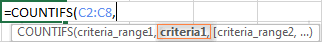### Excel COUNTIFS - things to remember!

1. You can use the COUNTIFS function in Excel to count cells in a single range with a single condition as well as in multiple ranges with multiple conditions. If the latter, only those cells that meet all of the specified conditions are counted.
2. Each additional range must have the same number of rows and columns as the first range (criteria_range1 argument).
3. Both contiguous and non-contiguous ranges are allowed.
4. If the criteria is a reference to an empty cell, the COUNTIFS function treats it as a zero value (0).
5. You can use the wildcard characters in criteria - asterisk (*) and question mark (?). See this example for full details.

## How to use COUNTIFS and COUNTIF with multiple criteria in Excel

Below you will find a number of formula examples that demonstrate how to use the COUNTIFS and COUNTIF functions in Excel to evaluate multiple conditions.

### How to count cells with multiple criteria (AND logic)

This scenario is the easiest one, since the COUNTIFS function in Excel is designed to count only those cells for which all of the specified conditions are TRUE. We call it the AND logic, because Excel's AND function works this way.

#### Formula 1. COUNTIFS formula with multiple criteria

Suppose you have a product list like shown in the screenshot below. You want to get a count of items that are in stock (value in column B is greater than 0) but have not been sold yet (value is column C is equal to 0).

The task can be accomplished by using this formula:

`=COUNTIFS(B2:B7,">0", C2:C7,"=0")`

And the count is 2 ("Cherries" and "Lemons"):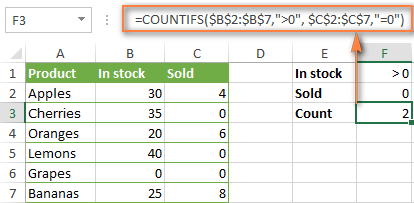#### Formula 2. COUNTIFS formula with the same criteria

When you want to count items with identical criteria, you still need to supply each criteria_range / criteria pair individually.

For example, here's the right formula to count items that have 0 both in column B and column C:

`=COUNTIFS(\$B\$2:\$B\$7,"=0", \$C\$2:\$C\$7,"=0")`

This COUNTIFS formula returns 1 because only "Grapes" have "0" value in both columns.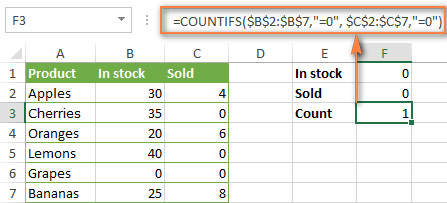Using a simpler formula with a single criteria_range like COUNTIFS(B2:C7,"=0") would yield a different result - the total count of cells in the range B2:C7 containing a zero (which is 4 in this example).

### How to count cells with multiple criteria (OR logic)

As you have seen in the above examples, counting cells that meet all of the specified criteria is easy because the COUNTIFS function is designed to work this way.

But what if you want to count cells for which at least one of the specified conditions is TRUE, i.e. based on the OR logic? Overall, there are two ways to do this - by adding up several COUNTIF formulas or using a SUM COUNTIFS formula with an array constant.

#### Formula 1. Add up two or more COUNTIF or COUNITFS formulas

In the table below, supposing you want to count orders with the "Cancelled" and "Pending" status. To have it doen, you can simply write 2 regular Countif formulas and add up the results:

`=COUNTIF(\$C\$2:\$C\$11,"Cancelled") + COUNTIF(\$C\$2:\$C\$11,"Pending")`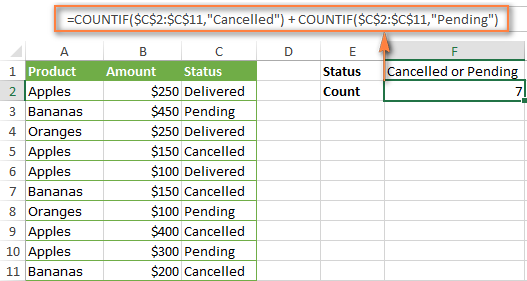In case each of the functions is supposed to evaluate more than one condition, use COUNTIFS instead of COUNTIF. For example, to get the count of "Cancelled" and "Pending" orders for "Apples" use this formula:

`=COUNTIFS(\$A\$2:\$A\$11, "Apples", \$C\$2:\$C\$11,"Cancelled") + COUNTIFS(\$A\$2:\$A\$11, "Apples", \$C\$2:\$C\$11,"Pending")`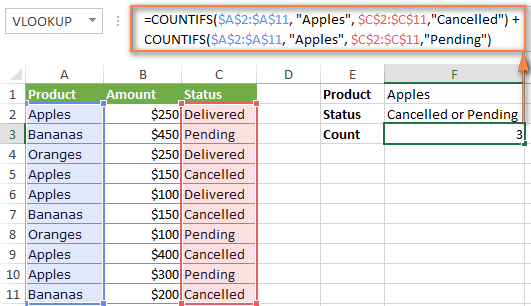#### Formula 2. SUM COUNTIFS with an array constant

In situations when you have to evaluate a lot of criteria, the above approach is not the best way to go because your formula would grow too big in size. To perform the same calculations in a more compact formula, list all of your criteria in an array constant, and supply that array to the criteria argument of the COUNTIFS function. To get the total count, embed COUNTIFS inside the SUM function, like this:

SUM(COUNTIFS(range,{"criteria1","criteria2","criteria3",…}))

In our sample table, to count orders with the status "Cancelled" or "Pending" or "In transit", the formula would go as follows:

`=SUM(COUNTIFS(\$C\$2:\$C\$11, {"cancelled", "pending", "in transit"}))`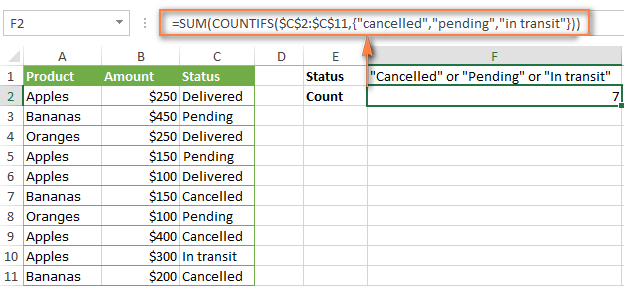In a similar manner, you can count cells based on two or more criteria_range / criteria pairs. For instance, to get the number of "Apples" orders that are "Cancelled" or "Pending" or "In transit", use this formula:

`=SUM(COUNTIFS(\$A\$2:\$A\$11,"apples",\$C\$2:\$C\$11,{"cancelled","pending","in transit"}))`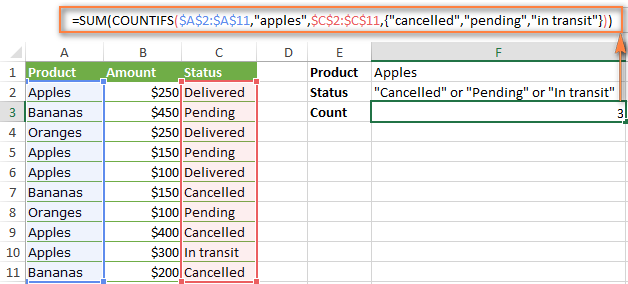You can find a few more ways to count cells with OR logic in this tutorial: Excel COUNTIF and COUNTIFS with OR conditions.

### How to count numbers between 2 specified numbers

By and large, COUNTIFS formulas for numbers fall into 2 categories - based on several conditions (explained in the above examples) and between the two values you specify. The latter can be accomplished in two ways - by using the COUNTIFS function or by subtracting one COUNTIF from another.

#### Formula 1. COUNTIFS to count cells between two numbers

To find out how many numbers between 5 and 10 (not including 5 and 10) are contained in cells C2 through C10, use this formula:

=COUNTIFS(C2:C10,">5", C2:C10,"<10")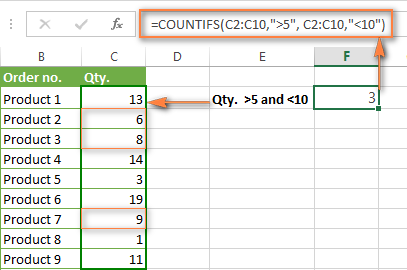To include 5 and 10 in the count, use the "greater than or equal to" and "less than or equal to" operators:

=COUNTIFS(B2:B10,">=5", B2:B10,"<=10")

#### Formula 2. COUNTIF formulas to count numbers between X and Y

The same result can be achieved by subtracting one Countif formula from another. The first one counts how many numbers are greater than the lower bound value (5 in this example). The second formula returns the count of numbers that are greater than the upper bound value (10 in this case). The difference between the first and second number is the result you are looking for.

• =COUNTIF(C2:C10,">5")-COUNTIF(C2:C10,">=10") - counts how many numbers greater than 5 and less than 10 are in the range C2:C10. This formula will return the same count as shown in the screenshot above.
• =COUNTIF(C2:C10, ">=5")-COUNTIF(C2:C10, ">10") - the formula counts how many numbers between 5 and 10 are in the range C2:C10, including 5 and 10.

### How to use cell references in COUNTIFS formulas

When using logical operators such as ">", "<", "<=" or ">=" together with cell references in your Excel COUNTIFS formulas, remember to enclose the operator in "double quotes" and
add an ampersand (&) before a cell reference to construct a text string.

In a sample dataset below, let's count "Apples" orders with amount greater than \$200. With criteria_range1 in cells A2:A11 and criteria_range2 in B2:B11, you can use this formula:

`=COUNTIFS(\$A\$2:\$A\$11, "Apples", \$B\$2:\$B\$11, ">200")`

Or, you can input your criteria values in certain cells, say F1 and F2, and reference those cells in your formula:

`=COUNTIFS(\$A\$2:\$A\$11, \$F\$1, \$B\$2:\$B\$11, ">"&\$F\$2)`

Please notice the use of absolute cell references both in the criteria and criteria_range arguments, which prevents the formula from being broken when copied to other cells.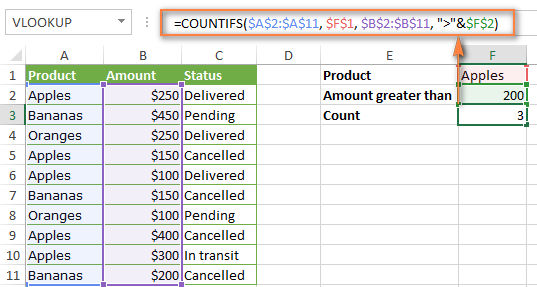### How to use COUNTIFS with wildcard characters

In Excel COUNTIFS formulas, you can use the following wildcard characters:

• Question mark (?) - matches any single character, use it to count cells starting and/or ending with certain characters.
• Asterisk (*) - matches any sequence of characters, you use it to count cells containing a specified word or a character(s) as part of the cell's contents.
Tip. If you want to count cells with an actual question mark or asterisk, type a tilde (~) before an asterisk or question mark.

Now let's see how you can use a wildcard char in real-life COUNTIFS formulas in Excel. Suppose, you have a list of projects in column A. You wish to know how many projects are already assigned to someone, i.e. have any name in column B. And because we are learning how to use the COUNTIFS function with multiple criteria, let's add a second condition - the End Date in column D should also be set.

Here is the formula that works a treat:

=COUNTIFS(B2:B10,"*",D2:D10,"<>"&""))

Please note, you cannot use a wildcard character in the 2nd criteria because you have dates rather that text values in column D. That is why, you use the criteria that finds non-blank cells: "<>"&""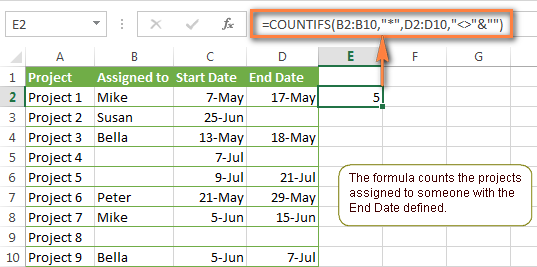### COUNTIFS and COUNTIF with multiple criteria for dates

The COUNTIFS and COUNTIF formulas you use for dates are very much similar to the above formulas for numbers.

#### Example 1. Count dates in a specific date range

To count the dates that fall in a certain date range, you can also use either a COUNTIFS formula with two criteria or a combination of two COUNTIF functions.

For example, the following formulas count the number of dates in cells C2 through C10 that fall between 1-Jun-2014 and 7-Jun-2014, inclusive:

`=COUNTIFS(C2:C9, ">=6/1/2014", C2:C9, "<=6/7/2014")`

`=COUNTIF(C2:C9, ">=6/1/2014") - COUNTIF(C2:C9, ">6/7/2014")`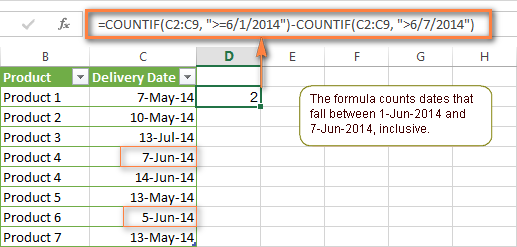#### Example 2. Count dates with multiple conditions

In the same manner, you can use a COUNTIFS formula to count the number of dates in different columns that meet 2 or more conditions. For instance, the below formula will find out how many products were purchased after the 20th of May and delivered after the 1st of June:

`=COUNTIFS(C2:C9, ">5/1/2014", D2:D9, ">6/7/2014")`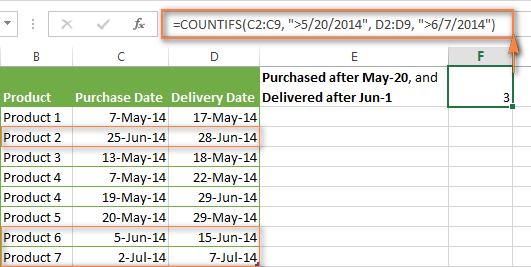#### Example 3. Count dates with multiple conditions based on the current date

You can use Excel's TODAY() function in combination with COUNTIF to count dates based on the current date.

For example, the following COUNTIF formula with two ranges and two criteria will tell you how many products have already been purchased but not delivered yet.

`=COUNTIFS(C2:C9, "<"&TODAY(), D2:D9, ">"&TODAY())`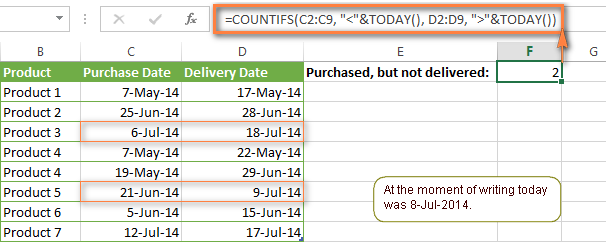This formula allows for many possible variations. For instance, you can tweak it to count how many products were purchased more than a week ago and are not delivered yet:

`=COUNTIFS(C2:C9, "<="&TODAY()-7, D2:D9, ">"&TODAY())`

This is how you count cells with multiple criteria in Excel. I hope you will find these examples helpful. Anyway, I thank you for reading and hope to see you on our blog next week!

## You may also be interested inWar in Ukraine! To support Ukraine and save lives please visit this page.